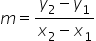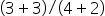Mathematics
Easy

Question

# Write the equation in point-slope form for the line that contains the points(-2, -3), (4, 3)

## y - 3 = 1(x - 2)y + 3 = 1(x + 2)y -3 = -1(x + 2)y + 3 = -1(x - 2)Hint:

## The correct answer is: y + 3 = 1(x + 2)

### Given points are (-2, -3), (4, 3)Step 1 : calculating SlopeSlope==1Step 2 : Point-slope form of equation of a line is y - y1 = m (x - x1)A line that passes through point (-2, -3) and has slope 1 isy + 3 = 1(x + 2) , is the equation of the line .

Point-slope form of equation of a line is y - y1 = m (x - x1)

### Related Questions to study#### With Turito Foundation.#### Get an Expert Advice From Turito.